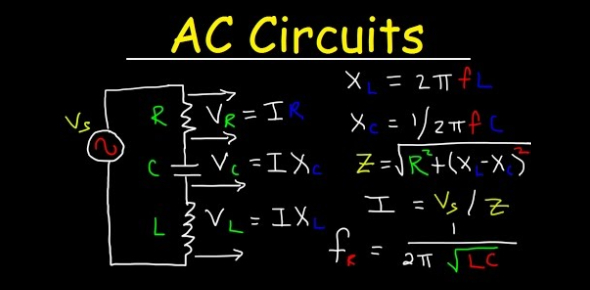# Interested In Electronics? Try This Quiz About AC Circuits

40 Questions | Total Attempts: 410SettingsThe circuit that is excited using alternating source is called an AC Circuit. The alternating current (AC) is used for domestic and industrial purposes. In an AC circuit, the value of the magnitude and the direction of current and voltages is not constant, it changes at a regular interval of time. Interested in Electronics? Try this Quiz about AC Circuits

• 1.
What is the frequency of a waveform that repeats 10 times in a half second ?
• A.

10 Hz

• B.

5 Hz

• C.

500 hz

• D.

.5 Hz

• E.

.1 Hz

• 2.
Inductive reactance is measured in what ?
• A.

Impedance

• B.

Z

• C.

Series

• D.

Parallel

• E.

Ohms

• 3.
RMS power is ?
• A.

Average power

• B.

True power

• C.

Apparent power

• D.

Distributed power

• E.

Peak to Peak power

• 4.
How does DC react to a capacitor ?
• A.

• B.

Capacitance rises

• C.

It is blocked

• D.

Phase shift

• E.

Leakage

• 5.
How many alternations form a cycle ?
• A.

1

• B.

2

• C.

3

• D.

4

• E.

6

• 6.
Inductors in series are the same as ?
• A.

Resistors parallel

• B.

Capacitors is series

• C.

Overall impedance

• D.

Resistors in series

• E.

Lambda

• 7.
What is the wavelength of a waveform ?(Multiple)
• A.

The times per second a waveform repeats

• B.

Time for a waveform to complete one cycle

• C.

Used to average a waveform

• D.

The distance traveled by a waveform during one second

• E.

The Lambda of a waveform

• 8.
The portion of the apparent power caused by inductors and capacitors ?
• A.

Average power

• B.

Apparent power

• C.

Reactive power

• D.

True power

• E.

The power factor

• 9.
In a capacitance circuit what is the relationship of voltage and current ?
• A.

• B.

There is a phase shift

• C.

• D.

They are in phase

• E.

Reactance is created

• 10.
In an AC circuit with only resistance the current is ?
• A.

Always in phase with the voltage

• B.

180 out of phase with voltage

• C.

Leads the voltage by 180 degrees

• D.

Lags behind the voltage by 180 degrees

• E.

Peaked out

• 11.
In a capacitive circuit the charge and discharge current must ?
• A.

Be simultaneous

• B.

Be in one direction

• C.

Always be in different directions

• D.

Always be out of phase

• E.

Be in phase

• 12.
A Farad is a measurement of what ?
• A.

Inductance

• B.

Capacitance

• C.

Reactance

• D.

Resistance

• E.

Impedance

• 13.
The characteristic of an electrical circuit to oppose the change of current flow.
• A.

Resistance

• B.

Capacitance

• C.

Inductance

• D.

Impedance

• E.

Reactance

• 14.
Unlike a resistor there is no power dissipation in an ideal ?
• A.

Inductor

• B.

Circuit

• C.

Capacitor

• D.

Capacitance circuit

• E.

Dielectric

• 15.
A Henry is a measurement of what ?
• A.

Resitance

• B.

Capacitance

• C.

Impedance

• D.

Reactance

• E.

Impedance

• 16.
What is the formula for capacitance reactance ?
• A.

XC = f x c

• B.

XC = 6.28 x f x t

• C.

XC = 1 / 6.28 x f x c

• D.

XC = 6.28 x f x c

• E.

XC = Square root (XC squared + X squared)

• 17.
What is the period of a waveform with a frequency of 60hz ?
• A.

17 milliseconds

• B.

1.7 milliseconds

• C.

170 milliseconds

• D.

.17 seconds

• E.

1.7 seconds

• 18.
Capacitors in series are the same as ?
• A.

Inductors in parallel

• B.

Resistors in series

• C.

Total impedance

• D.

Reactance

• E.

Capacitance reactance

• 19.
Commercial power is always given in ?
• A.

Peak to Peak

• B.

Peak

• C.

Average

• D.

RMS

• E.

Periodic

• 20.
The power factor is relationship between what to figures ?
• A.

Average and true power

• B.

Reactance and average power

• C.

True and apparent power

• D.

Apparent and reactance power

• E.

Resistance and reactance

• 21.
In an inductive circuit what happens when frequency increases ?
• A.

No change

• B.

There is a reduction in inductance

• C.

There is an increase in inductance

• D.

There is an increase in inductive reactance

• E.

There is a decrease in inductive reactance

• 22.
What is the formula to find RMS voltage ?
• A.

RMS = Peak / .707

• B.

RMS = .707 x Peak

• C.

RMS = .637 x Peak

• D.

RMS = .077 x Peak

• E.

RMS = .7 X Peak

• 23.
On a current transformer which set of windings always has more turns ?
• A.

They are the same

• B.

The primary

• C.

The like-wound side

• D.

The secondary

• E.

The unlike-wound side

• 24.
How is a current transformer connected ?
• A.

In series

• B.

In parallel

• C.

In proximity

• D.

Across the differing phases

• E.

Wrapped

• 25.
The product of the source voltage and total current ?
• A.

Average power

• B.

Apparent power

• C.

Reactive power

• D.

True power

• E.

The power factor

Related TopicsBack to top